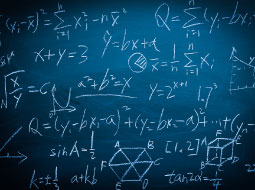# Algebra - Functions, Expressions and Equations

## Learn more about Algebra calculations, including expressions, equations, and variables, in a clear and simple manner.

Algebra
Free Course
Algebra is the foundation for all higher levels of math. It involves solving equations, isolating variables, and simplifying expressions. In a clear and simple manner, this course covers key algebra topics including expressions, equations, and variables. You will also study integers, rational numbers, square roots, scientific notations, and real numbers. You will then learn how to solve linear equations, visualize linear functions, and more.

2-3 Hours

Yes

Yes
• ### Publisher

Math Planet## Description

An algebraic expression is a mathematical phrase that can contains ordinary numbers, variables such as x or y, and operators such as add, subtract, multiply, and divide. This course begins by discussing expressions and variables. You will learn how to compose expressions, equations, and inequalities. You will then study distributive properties and learn how to solve ratios and proportions. You will also study how to calculate with percentages. This course will also discuss linear equations in the coordinate plane and the slope-intercept form of a linear equation.

You will then study parallel and perpendicular lines, scatter plots, and linear models. You will learn how to solve linear inequalities, compound inequalities, and absolute value equations and inequalities. This course will also teach you about graphing linear systems, the elimination method for solving linear systems, and the substitution method for solving linear systems. You will also look into the properties of exponents and exponential growth. You will then look into monomials, polynomials, special factors of polynomials, and the polynomial equation in factored form.

Algebra is an important life skill since it moves us beyond basic math and prepares us for statistics and calculus. Upon the completion of this course, you will be able to use graphing to solve quadratic equations, simplify radical equations, simplify and multiply rational expressions, add and subtract rational expressions, and solve rational expressions. So, check out the course today, and start making algebra a lot easier!

Start Course Now

## Learning Outcomes

This course will help you to understand expressions, equations and functions. You will be able to explore real numbers and solve linear equations, along with gaining a good knowledge of formulating linear equations and inequalities. You will know exponents and exponential functions. This course will help you to understand factoring, polynomials and how to formulate quadratic equations and radical expressions.

## Certification

All Alison courses are free to enrol, study and complete. To successfully complete this Certificate course and become an Alison Graduate, you need to achieve 80% or higher in each course assessment. Once you have completed this Certificate course, you have the option to acquire official Certification, which is a great way to share your achievement with the world. Your Alison Certification is:

Ideal for sharing with potential employers - include it in your CV, professional social media profiles and job applications
An indication of your commitment to continuously learn, upskill and achieve high results
An incentive for you to continue empowering yourself through lifelong learning

Alison offers 3 types of Certification for completed Certificate courses:

Digital Certificate - a downloadable Certificate in PDF format, immediately available to you when you complete your purchase
Certificate - a physical version of your officially branded and security-marked Certificate, posted to you with FREE shipping
Framed Certificate - a physical version of your officially branded and security-marked Certificate in a stylish frame, posted to you with FREE shipping